# Laser Pointer Panther Tank

Build a robotic Panther tank that can use a laser pointer to play with a cat! It also uses a custom controller with Bluetooth.

IntermediateFull instructions provided20 hours6,582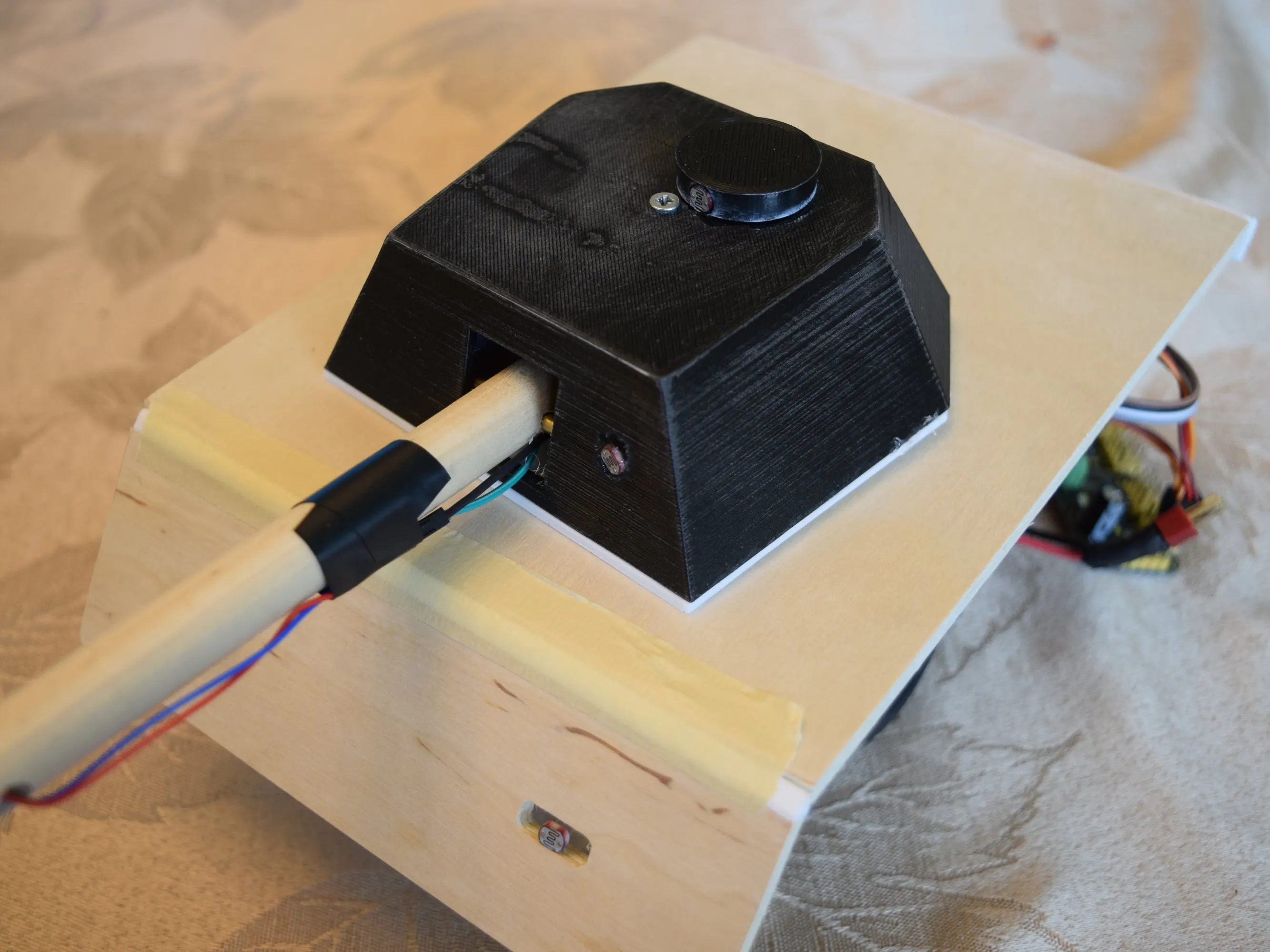## Things used in this project

### Hardware components

 DFRobot MiniQ Chassis
×1
 Laser Diode
×1
×1Texas Instruments Dual H-Bridge motor drivers L293D
×1
 5V Regulator
×2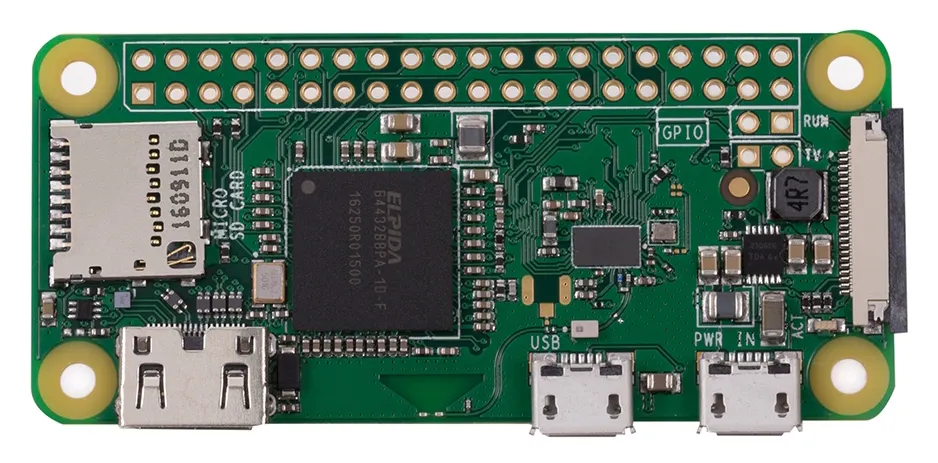Raspberry Pi Zero Wireless
×1
 9g Micro Servo
×2
 Joystick
×2
 LCD I2C
×1Arduino Nano R3
×1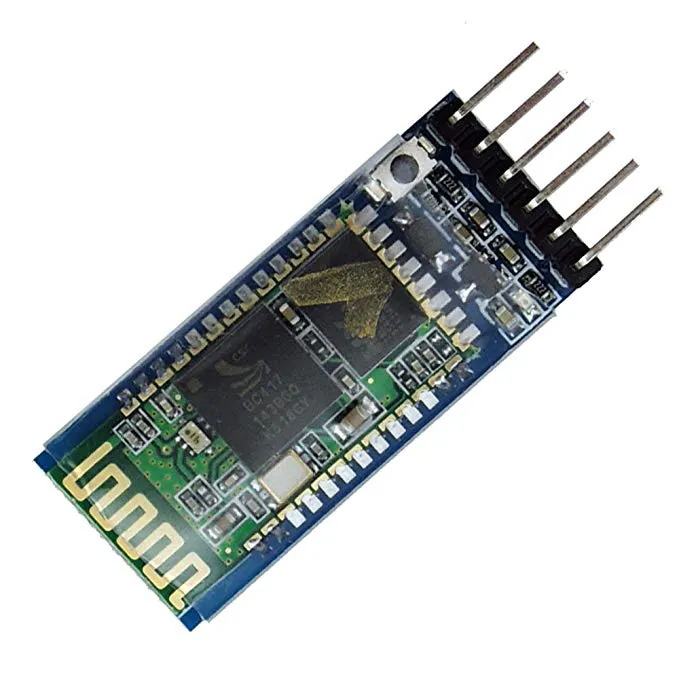HC-05 Bluetooth Module
×1LED (generic)
×4SparkFun Pushbutton switch 12mm Actually only 6mm
×1
 1800 mAh LiPo Battery 11.1V 30C
×1
 1300 mAh LiPo Battery 7.4V 30C
×1
 Transistor NPN
×1

### Software apps and online servicesArduino IDEAutodesk Fusion 360

### Hand tools and fabrication machines

 3D Printer I used the XYZ DaVinci ProSoldering iron (generic)

## Schematics

### Robot Schematic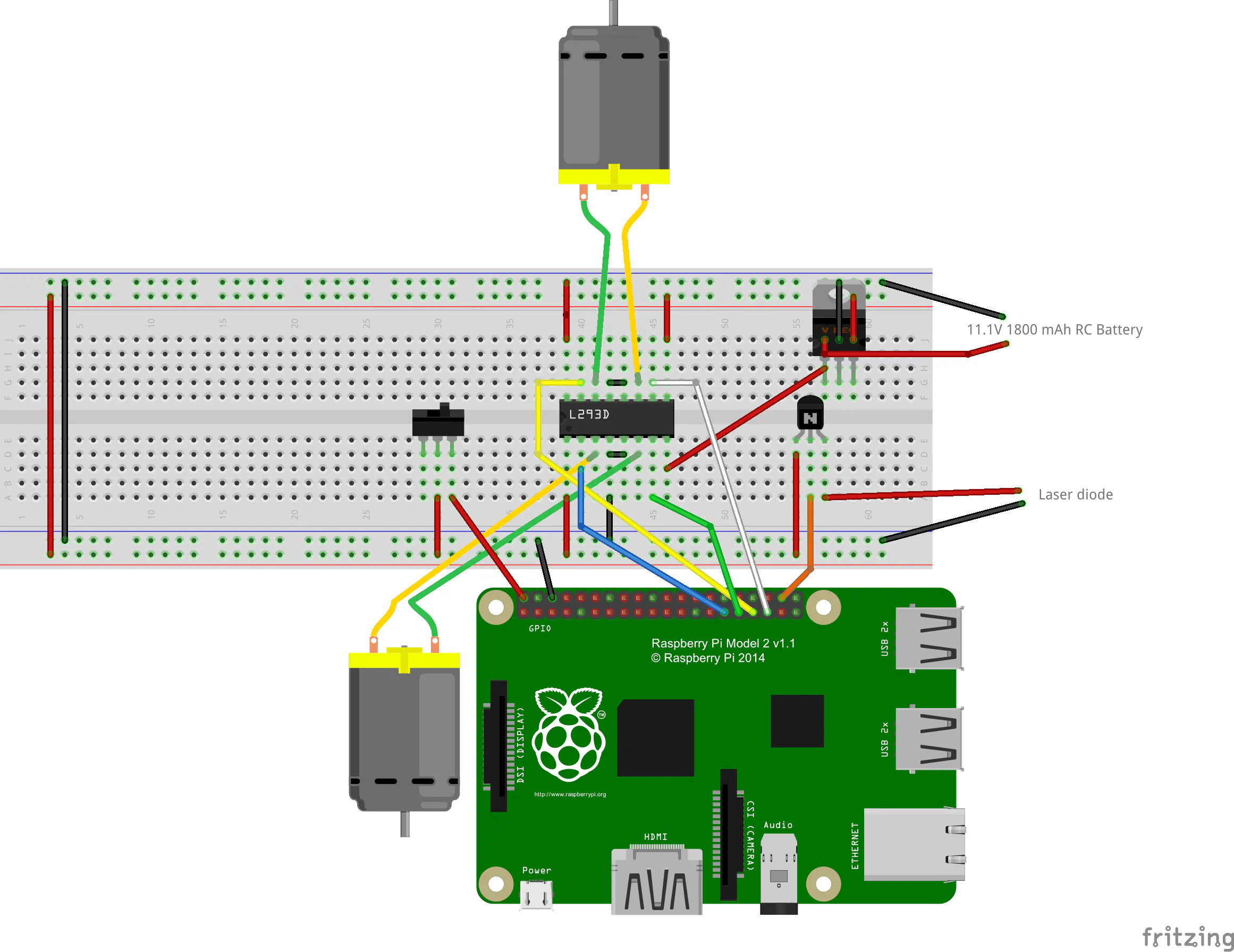### Controller Schematic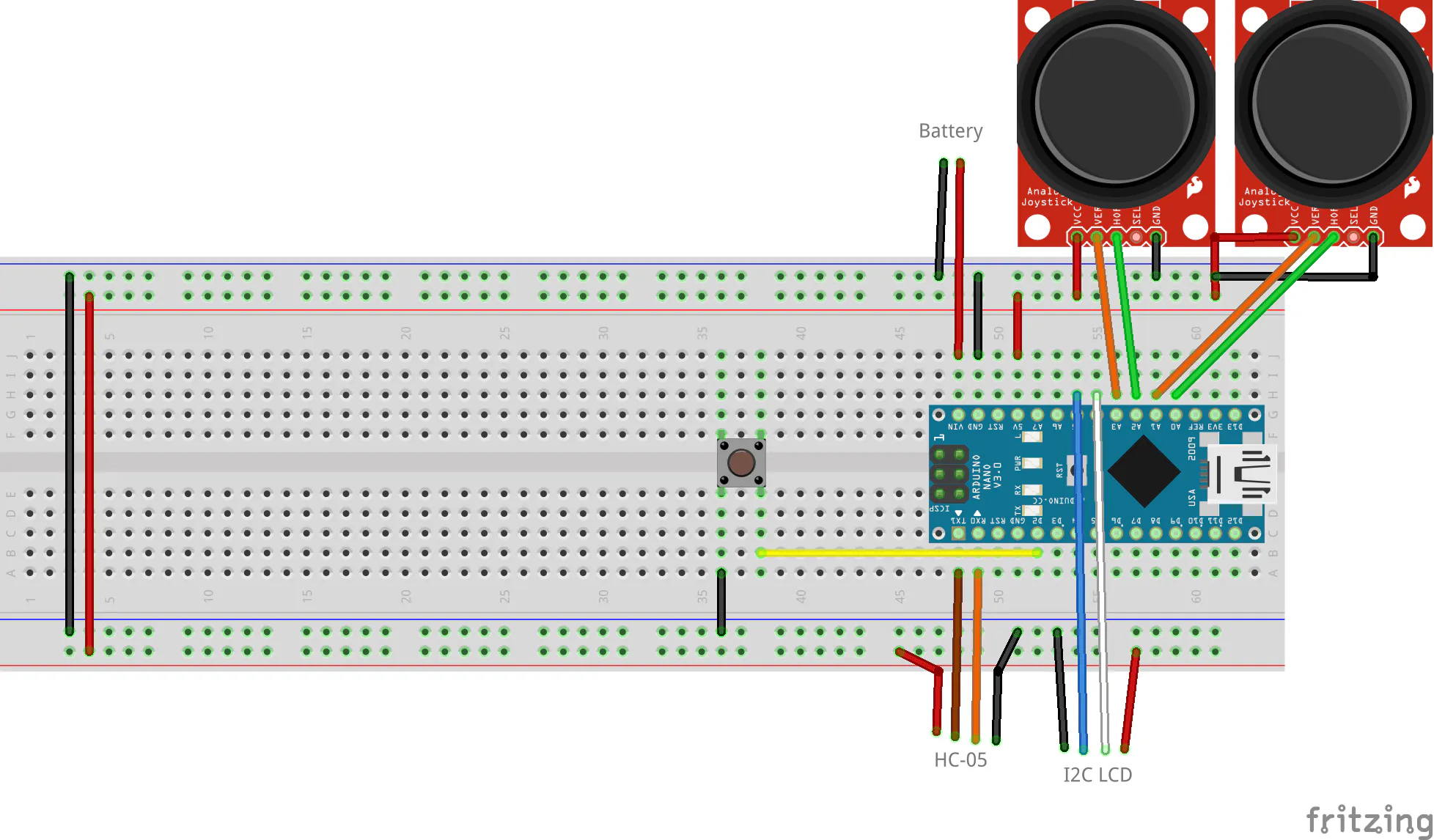## Code

### Controller Code

C/C++
```#include "Timer.h"

int JS_VALS = {};
int JS_VALS_R = {};

const int JS_PINS = {A0, A1, A2, A3}; //L_X, L_Y, R_X, R_Y

const int button_pin = 2;

const int servo_limits = {0, 180, 50, 95, 75}; //Left, Right, Lower, Upper, Middle limits for the turret
int turret_positions = {90, servo_limits}; //Initial positions

unsigned long last_time = 0;
long motor_delay = 30; //Delay 30 ms between motor control polls
long turret_delay = 150; //Delay 150 ms between turret control polls

Timer t;

void setup() {
// put your setup code here, to run once:
Serial.begin(9600);
pinMode(button_pin, INPUT_PULLUP);
attachInterrupt(digitalPinToInterrupt(button_pin), fire_laser, FALLING);
Serial.println("Begin");

t.every(motor_delay, control_motors);
t.every(turret_delay, control_turret);

}

void loop() {
/*control_motors();
control_turret();
delay(150);*/
t.update();

}

void fire_laser(){
String json_string = "{\"shoot\":\"1\"}";
Serial.println(json_string);
delay(10);
}

void control_motors(){
int x = map(JS_VALS, 0, 1023, -50, 50);
x = constrain(x, -100, 100);
int y = map(JS_VALS, 0, 1023, -50, 50);
y = constrain(y, -100, 100);
int motor1 = x+y;
int motor2 = y-x;
motor1 = constrain(motor1, -100, 100);
motor2 = constrain(motor2, -100, 100);
motor1 = 0;
}
motor2 = 0;
}
String json_string = "{\"motor\":[" + String(motor1)+","+String(motor2)+"]}";
Serial.println(json_string);
}

void control_turret(){
JS_VALS_R = analogRead(JS_PINS); //Get X values
JS_VALS_R = analogRead(JS_PINS); //Get Y values
int x = map(JS_VALS_R, 0, 1023, 5, -5);
x = constrain(x, -5, 5); //Catch outliers
int y = map(JS_VALS_R, 0, 1023, 2, -2);
y = constrain(y, -2, 2);

turret_positions += x;
turret_positions += y;

if(turret_positions <= servo_limits){
turret_positions = servo_limits;
}
else if(turret_positions >= servo_limits){
turret_positions = servo_limits;
}
if(turret_positions <= servo_limits){
turret_positions = servo_limits;
}
else if(turret_positions >= servo_limits){
turret_positions = servo_limits;
}

String json_string = "{\"turret\":[" + String(turret_positions)+","+String(turret_positions)+"]}";
Serial.println(json_string);

}
```

### Robot Class

Python
Put this in the same folder as "rc_car_main.py"
```from __future__ import division
import RPi.GPIO as GPIO
from time import sleep
from serial import Serial
import json

class Car(object):

def __init__(self, BT_Port, laser_pin):
GPIO.setmode(GPIO.BCM)
self.ser = Serial(BT_Port, baudrate=9600)
self.laser_pin = laser_pin
self.laser_state = False
GPIO.setup(self.laser_pin, GPIO.OUT)

def configMotors(self, mL1, mL2, mR1, mR2, invertLR=False):
self.motor_array = [mL1, mL2, mR1, mR2]
self.invert = invertLR
for pin in self.motor_array:
GPIO.setup(pin, GPIO.OUT)
#self.motor_pwm.append(GPIO.PWM(pin, 490))
self.motorL1_pwm = GPIO.PWM(mL1, 100)
self.motorL2_pwm = GPIO.PWM(mL2, 100)
self.motorR1_pwm = GPIO.PWM(mR1, 100)
self.motorR2_pwm = GPIO.PWM(mR2, 100)
self.motorL1_pwm.start(0)
self.motorL2_pwm.start(0)
self.motorR1_pwm.start(0)
self.motorR2_pwm.start(0)

def configServos(self):
self.servo_min = 150
self.servo_max = 600
self.pwm.set_pwm_freq(60)

def deg_to_pulse(self, degree):
pulse = (degree - 0) * (self.servo_max - self.servo_min) / (180 - 0) + 150
print('Pulse: '+str(pulse))
return int(pulse)

self.cmd = ""
if self.ser.inWaiting():
if self.cmd != None:
try:
data = self.cmd.decode('utf-8')
print data
if data != "":
return data
else:
return ""
except ValueError:
pass

def turn_wheel(self, motor1, motor2):
if self.invert:
temp = motor1
motor1 = motor2
motor2 = temp
motor1 = (motor1 / 2)
motor2 = (motor2 / 2)
if motor1<0:
self.motorL2_pwm.ChangeDutyCycle(motor1*-1)
self.motorL1_pwm.ChangeDutyCycle(0)#make positive if negative
else:
self.motorL1_pwm.ChangeDutyCycle(motor1)
self.motorL2_pwm.ChangeDutyCycle(0)

if motor2<0:
self.motorR2_pwm.ChangeDutyCycle(motor2*-1)
self.motorR1_pwm.ChangeDutyCycle(0)#make positive if negative
else:
self.motorR1_pwm.ChangeDutyCycle(motor2)
self.motorR2_pwm.ChangeDutyCycle(0)

def move_turret(self, turret_deg, barrel_deg):
self.pwm.set_pwm(0, 0, self.deg_to_pulse(turret_deg))
self.pwm.set_pwm(1, 0, self.deg_to_pulse(barrel_deg))

def fire_laser(self):
self.laser_state =  not self.laser_state
GPIO.output(self.laser_pin, self.laser_state)

def update(self): #Run to make the robot work
print self.json_obj
try:
if "motor" in self.cmd:
if self.json_obj["motor"] != None:
val1 = self.json_obj["motor"]
# if val1 > 0:
#    val1 += 10
val2 = self.json_obj["motor"]
# if val2 <0:
#	val2 += 4
self.turn_wheel(val1, val2)
elif "shoot" in self.cmd:
self.fire_laser()
elif "turret" in self.cmd:
print('Turret found!!!!!!!!!!')
val1 = self.json_obj["turret"]
val2 = self.json_obj["turret"]
self.move_turret(val1, val2)

except TypeError:
pass
```

### Robot Main

Python
Run this with sudo python2 <filename>
```import car

bt_port = "/dev/rfcomm3"
laser_pin = 20
rc_car = car.Car(bt_port, laser_pin)

rc_car.configMotors(6,5,19,13, invertLR=True)
rc_car.configServos() #Turret drive servo, barrel servo

while 1:
rc_car.update()

```

## Credits

### Arduino “having11” Guy

70 projects • 443 followers
IoT and embedded systems enthusiast. Also an intern at Hackster.io who loves working on projects and sharing knowledge.
Thanks to AceofAces16.